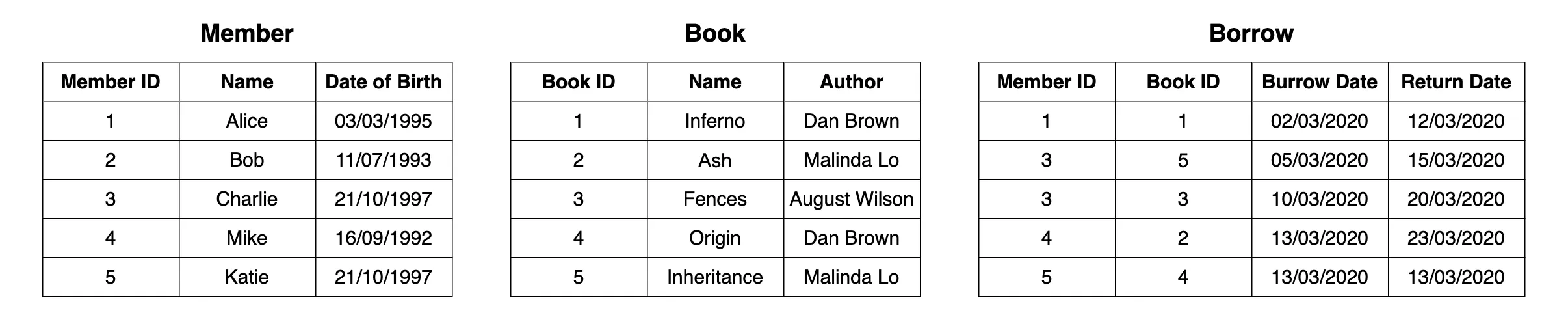# SQL and Relational Algebra# SQL and Relational Algebra

Relational algebra (RA) is considered as a procedural query language where the user tells the system to carry out a set of operations to obtain the desired results. i.e. The user tells what data should be retrieved from the database and how to retrieve it.

Relational algebra notions can be implemtned via any any SQL language like PL/SQL, TSQL, SQLite SQL, DB2 SQL, MariaDB SQL, FireBird SQL, PSQL, ANSI SQL commands in any databases like MySQL, PostgreSQL, Oracle, SQLServer SQL server.## 1. SELECT (σ) - Where clause

Used for selecting/filtering rows/tuples/records.

$$σ_p(r)$$ σ is the predicate r stands for relation which is the name of the table

p is prepositional logic

$$σ_{topic = 'Database'}(Tutorials)$$
$$σ_{topic = 'Database' and author = 'Hari'}( Tutorials)$$
$$σ_{sales > 50000} (Customers)$$

## 2. Projection(π) - Select Attributes

Used for selecting columns/attributes/features.

$Π_{CustomerName, Status} (Customers)$

## 3. Rename (ρ)

ρ (a/b)R will rename the attribute ‘b’ of relation by ‘a’.

$ρ (EmpName/EmployeeName)R$

## 4. Union operation (υ)

A ∪ B

For a union operation to be valid, the following conditions must hold –

A and B must be the same number of attributes. Attribute domains need to be compatible. Duplicate tuples should be automatically removed.

## 5. Set Difference (-)

A – B

The attribute name of A has to match with the attribute name in B. The two-operand relations A and B should be either compatible or Union compatible. It should be defined relation consisting of the tuples that are in relation A, but not in relation B.

## 6. Intersection

A ∩ B

The attribute name of A has to match with the attribute name in B. The two-operand relations A and B should be either compatible or Union compatible. It should be defined relation consisting of the tuples that are in relation A, and in relation B.

## 7. Cartesian Product(X) in DBMS

The example shows all rows from relation A and B whose column 2 has value 1

$σ_{column 2 = '1'} (A X B)$

## 8. Join Operations

Join operation is essentially a cartesian product followed by a selection criterion.

Join operation denoted by ⋈.

### Inner Joins:

In an inner join, only those tuples that satisfy the matching criteria are included, while the rest are excluded

#### 8.1 Theta: A ⋈θ B

• $A ⋈ _{A.column 2 > B.column 2} (B)$

#### 8.2 EQUI join

• $A ⋈ _{A.column 2 = B.column 2} (B)$

#### 8.3 Natural join :

Natural join can only be performed if there is a common attribute between the relations

• C ⋈ D

### 9. Outer join:

#### 9.1 Left Outer Join(A ⟕ B)

In the left outer join, operation allows keeping all tuple in the left relation.

#### 9.2 Right Outer Join ( A ⟖ B )

In the right outer join, operation allows keeping all tuple in the right relation

#### 9.3 Full Outer Join ( A ⟗ B)

In a full outer join, all tuples from both relations are included in the result irrespective of the matching condition.

Tags:

Updated: